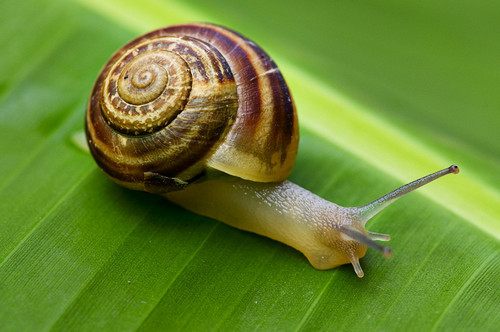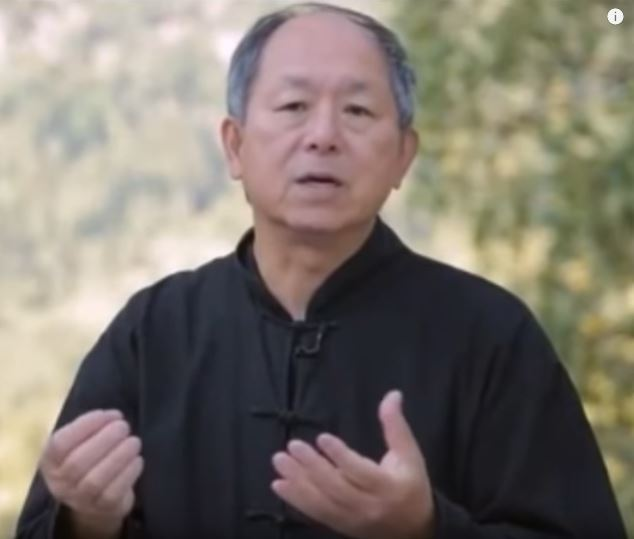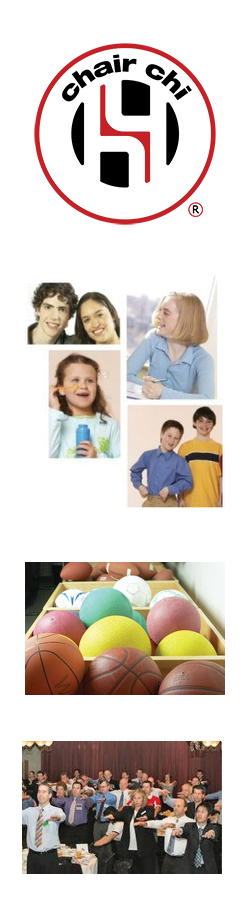Like   Tweet   Pin   +1   in
 /* styles */ Tai Chi Stories, Tips and Resources   ||   Issue #19 September 2019
 table div table+table+table div table{width:100%;padding:0}table div table+table+table div table img{width:96.23%;padding:0;float:none}table div table+table+table div table td{width:100%;padding:0 1.88% 18px}/* styles */table div table+table+table+table div table,table.module-3{width:3.77%;float:right;padding:0}table div table+table+table+table div table a{border:0 none;text-decoration:none}table div table+table+table+table div table img{width:100%!important;border:0 none;text-decoration:none}table div table+table+table+table div table td{width:100%;padding:0 0 20px 20px}/* styles */ Dear Reader, This month you can read about how one aged care resident eventually participated in my Chair Chi session. :) I share a video that gives a good explanation of Qi/Chi - which is something that can be difficult to explain - and there's a Tai Chi tip that I've found is actually good advice for most other things as well! You'll find part five of my series on What is Tai Chi Chuan, in which you can learn about Chi Kung. There's also a summary of all the services I offer for various sectors. Regards Chris Bennett Chris Chi
 table div table+table+table+table+table div table{width:100%;padding:0}table div table+table+table+table+table div table img{width:96.23%;padding:0;float:none}table div table+table+table+table+table div table td{width:100%;padding:0 1.88% 18px}/* styles */## Eddie's StoryEddie rarely moves while lying in his tub chair - he usually just watches or falls asleep during our Chair Chi sessions.

I always gently try to encourage him to participate, but for the past several sessions he had barely moved.

Then this day it happened!

 table div table+table+table+table+table+table+table+table div table{width:100%;padding:0}table div table+table+table+table+table+table+table+table div table img{width:96.23%;padding:0;float:none}table div table+table+table+table+table+table+table+table div table td{width:100%;padding:0 1.88% 18px}/* styles */## Go Slow Feel MoreThe long form in Wu Stye Tai Chi contains 37 postures, repeats of some of those postures, and transitions - it usually takes between 15-20 minutes to practice. If I'm busy, sometimes I tend to rush it and end up practicing for only 15 minutes. 15 minutes is a good workout, but 20 minutes is even better. *Read more ...*

 table div table+table+table+table+table+table+table+table+table+table+table div table{width:100%;padding:0}table div table+table+table+table+table+table+table+table+table+table+table div table img{width:96.23%;padding:0;float:none}table div table+table+table+table+table+table+table+table+table+table+table div table td{width:100%;padding:0 1.88% 18px}/* styles */## What Is QiMaster Yang Jwing Ming

Here's a short, scientific explanation of Qi (Chi) by Master Yang Jwing Ming. Explaining Chi to the layperson can be difficult, and it's always handy to watch clips like this to get a basic understanding.

I have several books on Chi in my library and research continually to get a deeper understanding myself. But understanding Chi is only the first step - the next one is to feel the Chi as you practice your Tai Chi and/or Chi Kung

 table div table+table+table+table+table+table+table+table+table+table+table+table+table+table div table{width:100%;padding:0}table div table+table+table+table+table+table+table+table+table+table+table+table+table+table div table img{width:96.23%;padding:0;float:none}table div table+table+table+table+table+table+table+table+table+table+table+table+table+table div table td{width:100%;padding:0 1.88% 18px}/* styles */table div table+table+table+table+table+table+table+table+table+table+table+table+table+table+table+table div table{width:100%;padding:0}table div table+table+table+table+table+table+table+table+table+table+table+table+table+table+table+table div table img{width:96.23%;padding:0;float:none}table div table+table+table+table+table+table+table+table+table+table+table+table+table+table+table+table div table td{width:100%;padding:0 1.88% 18px}/* styles */## What is Tai Chi Chuan? Part Five - Chi Kung/Nei King

 /* styles */ Tai Chi Chuan is a health and self defence system. There are various styles of Tai Chi and the major styles are Yang, Wu, Chen, Sun, Wu Hao. Each of these styles comprises five sections: The Hand Form; Push Hands; Chi Kung/Nei Kung; Self Defence; and Weapons (sword, sabre, spear). I've included Chi Kung as part of Tai Chi because of the shared commonality of the concept of Chi.
 table div table+table+table+table+table+table+table+table+table+table+table+table+table+table+table+table+table+table+table div table,table.module-18{width:28.3%;float:right;padding:0}table div table+table+table+table+table+table+table+table+table+table+table+table+table+table+table+table+table+table+table div table a{border:0 none;text-decoration:none}table div table+table+table+table+table+table+table+table+table+table+table+table+table+table+table+table+table+table+table div table img{width:100%!important;border:0 none;text-decoration:none}table div table+table+table+table+table+table+table+table+table+table+table+table+table+table+table+table+table+table+table div table td{width:100%;padding:0 0 20px 20px}/* styles */ There are many sets of Qigong, the most popular being The Eight Treasures (sometimes called the The Eight Brocades) emphasising health and well being. There are slight variations in the Eight Treasures; it depends on who is teaching the set. Here's one way of performing the Eight treasures.
 /* styles */ Nei Kung is internal strength training of Tai Chi and is usually taught to "indoor students" and not to the general public. Because of the secrecy around nei kung training, I've included an example of what it can do. This requires specialist training, so please do not attempt what's in this video clip!

Tai Chi Chuan Nei Kung demonstration - Cloud Dragon school

## Previous Issues

Part One - Hand Form - May Issue
Part Two - Weapons - June Issue
Part Three - Push Hands - July Issue
Part Four - Self Defence - August Issue

 table div table+table+table+table+table+table+table+table+table+table+table+table+table+table+table+table+table+table+table+table+table+table div table{width:100%;padding:0}table div table+table+table+table+table+table+table+table+table+table+table+table+table+table+table+table+table+table+table+table+table+table div table img{width:96.23%;padding:0;float:none}table div table+table+table+table+table+table+table+table+table+table+table+table+table+table+table+table+table+table+table+table+table+table div table td{width:100%;padding:0 1.88% 18px}/* styles */# Tai Chi Tip

Focus on continuous improvement; not comparisons with others.

 table div table+table+table+table+table+table+table+table+table+table+table+table+table+table+table+table+table+table+table+table+table+table+table+table div table{width:100%;padding:0}table div table+table+table+table+table+table+table+table+table+table+table+table+table+table+table+table+table+table+table+table+table+table+table+table div table img{width:96.23%;padding:0;float:none}table div table+table+table+table+table+table+table+table+table+table+table+table+table+table+table+table+table+table+table+table+table+table+table+table div table td{width:100%;padding:0 1.88% 18px}/* styles */## Summary of My ServicesI get around a bit and here's a summary of all the presentations, workshops and programs I run for various sectors.

## Sport

Sports Chi for players

## Organisations

Conference/Meeting Energizers

 table div table+table+table+table+table+table+table+table+table+table+table+table+table+table+table+table+table+table+table+table+table+table+table+table+table+table+table div table{width:100%;padding:0}table div table+table+table+table+table+table+table+table+table+table+table+table+table+table+table+table+table+table+table+table+table+table+table+table+table+table+table div table img{width:96.23%;padding:0;float:none}table div table+table+table+table+table+table+table+table+table+table+table+table+table+table+table+table+table+table+table+table+table+table+table+table+table+table+table div table td{width:100%;padding:0 1.88% 18px}/* styles *//* styles */